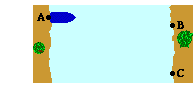## Riverboat Simulator Activity Sheet

### Purpose:

The purpose of this activity is to analyze the relationship between the two vector components of motion for a river boat as it travels across a river in the presence of a current.

### Procedure and Questions:

1. Navigate to the Riverboat Simulator page and experiment with the on-screen buttons in order to gain familiarity with the control of the animation. The width of the river, speed of the river, speed of the boat, and direction (or heading) of the boat can be modified. The animation can be started, paused, continued, single-stepped or rewound.

After gaining familiarity with the program, use it to answer the following questions:

2. Will a change in the speed of a current change the time required for a boat to cross a 100 m wide river? _________ In the space below, display some collected data which clearly support your answer. Discuss how your data provide support for your answer.

3. For a constant river width and boat heading, what variable(s) effects the time required to cross a 100 m wide river? __________________________ In the space below, display some collected data which support your answer. Discuss how your data provide support for your answer.

4. Suppose that a motor boat can provide a maximum speed of 10 m/s with respect to the water. What heading will minimize the time for that boat to cross a 100-m wide river? ___________ In the space below, display some collected data which clearly support your answer. If necessary, discuss how your data provide support for your answer.

5. Run the simulation with the following combinations of boat speeds and current speeds with a heading of 0 degrees (due East). Before running each simulation, perform quick calculations to determine the time required for the boat to reach the opposite bank (of a 100-meter wide river) and the distance that the boat will be carried downstream by the current. Use the simulation to check your answer(s).

 Boat Speed (m/s) Current Speed (m/s) Time to CrossRiver (s) Distance Downstream (m) 12 2 12 3 12 4 20 2 20 5

6. Study the results of your calculations in the table above and answer the following two questions.

• What feature in the table above is capable of changing the time required for the boat to reach the opposite bank of a 100-meter wide river? Explain.
• What two quantities are needed to calculate the distance the boat travels downstream?

7. Use what you have learned from the distance-speed-time relationships to solve the following two problems.

A waterfall is located 45.0 m downstream from where the boat is launched. If the current speed is 3 m/s, then what minimum boat speed is required to cross the 100-meter wide river before falling over the falls? Show your calculations and then check your prediction using the simulation.

Repeat the above calculations to determine the boat speed required to cross the 100-meter wide river in time if the current speed was 5 m/s and the waterfall was located 45.0 m downstream. Again, check your predictions using the simulation. PSYWFor Questions 8 and 9: Consider a boat which begins at point A and heads straight across a 100-meter wide river with a speed of 8 m/s (relative to the water). The river water flows south at a speed of 3 m/s (relative to the shore). The boat reaches the opposite shore at point C.

8. Which of the following would cause the boat to reach the opposite shore in MORE time? Circle all that apply.

a. The river is 80 meters wide.
b. The river is 120 meters wide.
c. The boat heads across the river at 6 m/s.
d. The boat heads across the river at 10 m/s.
e. The river flows south at 2 m/s.
f. The river flows south at 4 m/s.
g. Nonsense! None of these effect the time to cross the river.

9. Which of the following would cause the boat to reach the opposite shore at a location SOUTH of C? Circle all that apply.

a. The boat heads across the river at 6 m/s.
b. The boat heads across the river at 10 m/s.
c. The river flows south at 2 m/s.
d. The river flows south at 4 m/s.
e. Nonsense! None of these effect the location where the boat lands.

10. Observe that the resultant velocity (v) is the vector sum of the boat velocity (vx) and the river velocity (vy). Use the principles of vector addition to determine the resultant velocity for each combination of boat/current velocities listed below. Use a sketch of the two vectors and the resultant accompanied by the use of the Pythagorean theorem and trigonometric functions to determine the magnitude and direction of the resultant. PSYW

 Boat Velocity = 15 m/s, East Current Velocity = 4 m/s, South Boat Velocity = 20 m/s, East Current Velocity = 5 m/s, South vresultant Magnitude: ___________________ m/s Direction: ________________ vresultant Magnitude: ___________________ m/s Direction: ________________

For the two sets of boat and current velocities listed above, use the Pythagorean theorem to calculate the resultant displacement of the boat in order to cross the 190-meter wide river. Show your calculations for each case in the space below.

 Boat Velocity = 15 m/s, East Current Velocity = 4 m/s, South dacross = 190 m Boat Velocity = 20 m/s, East Current Velocity = 5 m/s, South dacross = 190 m ddownstream = _____________ dresultant = _____________ ddownstream = _____________ dresultant = _____________

### Summary Statement:

It is often said that perpendicular components of motion are independent of each other. Explain the meaning of this statement and apply it to the motion of a river boat in the presence of a current.

Start Activity